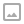## 「JSOI2016」最佳团体-树上背包+0/1分数规划

·  ✏️ About  690 words  ·  ☕ 2 mins read · 👀... views

JSOI 信息学代表队一共有 $N$ 名候选人，这些候选人从 $1$ 到 $N$ 编号。方便起见，JYY 的编号是 $0$ 号。每个候选人都由一位编号比他小的候选人$R_i$推荐。如果 $R_i = 0$ ，则说明这个候选人是 JYY 自己看上的。

Luogu P4322

## 代码

0/1分数规划的过程，就是二分选择一个答案 $ans$ ，然后去验证能不能取到若干个 $P_i - ans \times S_i$ 大于0。

## 代码

  1 2 3 4 5 6 7 8 9 10 11 12 13 14 15 16 17 18 19 20 21 22 23 24 25 26 27 28 29 30 31 32 33 34 35 36 37 38 39 40 41 42 43 44 45 46 47 48 49 50 51 52 53 54 55 56 57 58 59 60 61 62  #include #include #include using namespace std; const int MAXN = 3000; const double eps = 1e-4; int n,m; double s[MAXN],p[MAXN]; int siz[MAXN]; double dp[MAXN][MAXN]; vector edge[MAXN]; double k; double tmp[MAXN]; void dfs(int x){ for(int j = 0;j < MAXN;j++) dp[x][j] = -1e9; siz[x] = 1;dp[x] = 0;dp[x] = p[x] - k*s[x]; for(int i = 0;i= 1;--j) for(int w = siz[v];w >= 0;--w) if(j+w <= m) tmp[j+w] = max(tmp[j+w],dp[x][j] + dp[v][w]); memcpy(dp[x],tmp,sizeof(double)*(siz[x] + siz[v]+1)); siz[x] += siz[v]; } } bool check(double num){ k = num; dfs(0); return dp[m] > -eps; } int main(){ scanf("%d %d",&m,&n);m++; int f; for(int i = 1;i<=n;i++){ scanf("%lf %lf %d",&s[i],&p[i],&f); edge[f].push_back(i); } double l = 0,r = 10000; while(r - l > eps){ double mid = (l+r)/2; if(check(mid)) l = mid; else r = mid; } printf("%.3lf\n",l); return 0; }WRITTEN BY
cqqqwq
A student in Computer Science.